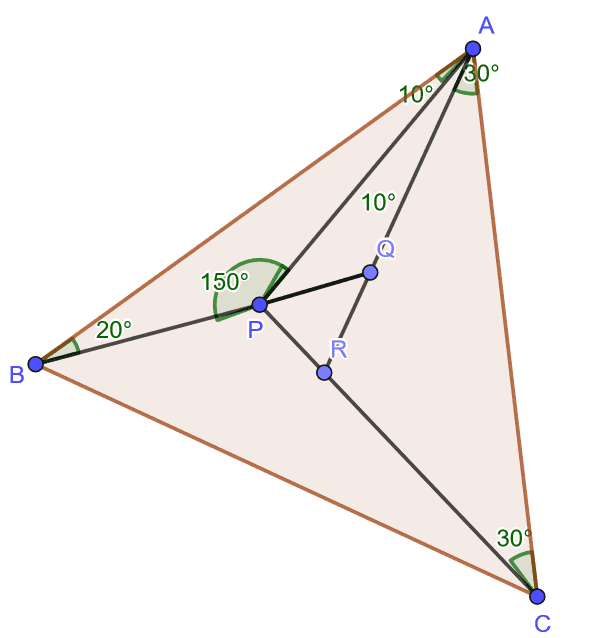# Prove that △ABC is isosceles.

• MHB
Gold Member
MHB
POTW Director
Triangle $ABC$ has the following property: there is an interior point $P$ such that $\angle PAB=10^{\circ},\, \angle PBA=20^{\circ}, \angle PCA=30^{\circ}$, and $\angle PAC = 40^{\circ}$. Prove that triangle $ABC$ is isosceles.

Gold Member
MHB
POTW Director
My solution:Let

i. $\triangle ABQ$ be an isosceles triangle where $\angle QAB=\angle QBA=20^{\circ}$ and the points $B, P$ and $Q$ are collinear and
ii. $\triangle ACR$ be another isosceles triangle where $\angle RAC=\angle RCA=30^{\circ}$ and the points $C, R$ and $P$ are collinear.

Consider $\triangle ABQ$, by applying the Sine Rule, we get
$\dfrac{AB}{\sin 140^{\circ}}=\dfrac{BQ}{\sin 20^{\circ}}\implies AB=2BQ\cos 20^{\circ}$

Now, in order to relate $AC$ in terms of $BQ$, we do the following:
$\dfrac{AC}{\sin 120^{\circ}}=\dfrac{AR}{\sin 30^{\circ}}\\ \dfrac{AR}{\sin 110^{\circ}}=\dfrac{AP}{\sin 60^{\circ}}\\ \dfrac{AP}{\sin 140^{\circ}}=\dfrac{AQ}{\sin 30^{\circ}}\\ \implies AC=\dfrac{\sin 120^{\circ}}{\sin 30^{\circ}}\dfrac{\sin 110^{\circ}}{\sin 60^{\circ}}\dfrac{\sin 140^{\circ}}{\sin 30^{\circ}}AQ=4\sin 70^{\circ}\sin 40^{\circ}BQ=4\cos 20^{\circ}\sin 40^{\circ}BQ$

Last, we consider $\triangle ABC$, by applying the Cosine Rule, we get
\begin{align*}BC^2&=AB^2+AC^2-2ABAC\cos 50^{\circ}\\&=(2BQ\cos 20^{\circ})^2+(4\cos 20^{\circ}\sin 40^{\circ}BQ)^2-2(2BQ\cos 20^{\circ})(4\cos 20^{\circ}\sin 40^{\circ}BQ)\sin 40^{\circ}\\&=4BQ^2\cos^2 20^{\circ}(1+4\sin^2 40^{\circ}-4\sin^2 40^{\circ})\end{align*}\\ \therefore BC=2BQ\cos 20^{\circ}

Hence, we have proved that $AB=BC\ne AC$ and that $\triangle ABC$ is isosceles.

Remark: I apologize for attaching a triangle that is so lacking in the labeling of the measure of angles. I wanted to use TiKZ to render my triangle initially but since we have disabled it (temporarily) in order to fix some other technical issue, and that I thought it was time to post solution to this challenge by now, it left me no choice but have to go ahead to use the far less than satisfactory diagram. But I hope it does justice to its purpose and let you make sense of my argument.

Last edited: